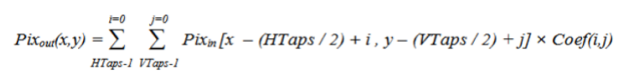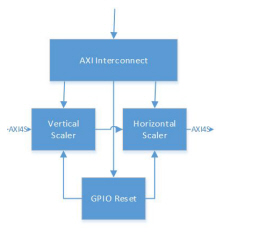# Scaling - 2.4 English

## Video Processing Subsystem Product Guide (PG231)

Document ID
PG231
Release Date
2022-10-19
Version
2.4 English

Video scaling is the process of converting an input color image of dimensions Xin pixels by Yin lines to an output color image of dimensions Xout pixels by Yout lines. The IP converts a specified rectangular area of an input digital video image from the original sampling grid to a desired target sampling grid.

The Scaler only mode runs in stream mode, where memory is not required. Any scaling ratio should work in the streaming mode as long as you ensure that the stream has properly dimensioned the performance of the scaler, by choosing pixels per clock and frequency. This is related to the maximum of the input and output resolution.

For example, in case of downscaling choose the maximum input resolution, and in case of upscaling choose the maximum output resolution. Resolution times frame rate determines the pixel throughput. You need to make sure that the scaler is configured to support this pixel throughput plus a margin of at least 10%. That is, when you want to upscale 1080p60 to 4K60, the required throughput is  = 4320 * 2160 * 60 (maximum of i/p and o/p resolution is 4k60)  = 530 MHz + 10% margin =~ 600 MHz. So, Scaler needs to be configured for 300 MHz input streaming clock and pixels per clock value as 2.

The input image must be provided in raster scan format (left to right and top to bottom). The valid outputs are also given in this order.

Video scaling is a form of 2-D filter operation which can be approximated with the equation shown in This Equation .

Equation 3-1In this equation, x and y are discrete locations on a common sampling grid; Pix out (x, y) is an output pixel that is being generated at location (x, y) ; Pix in (x, y) is an input pixel being used as part of the input scaler aperture; Coef (i, j) is an array of coefficients that depend upon the application; and HTaps and VTaps are the number of horizontal and vertical taps in the filter, respectively.

The coefficients in this equation represent weights applied to the set of input samples chosen to contribute to one output pixel, according to the scaling ratio. Scaler only mode requires both external and internal gpio reset to be asserted, when there is a change in input resolution.

 X-Ref Target - Figure 3-3